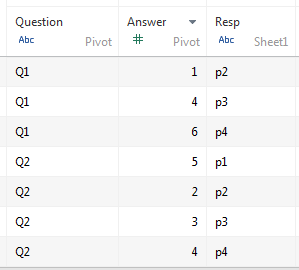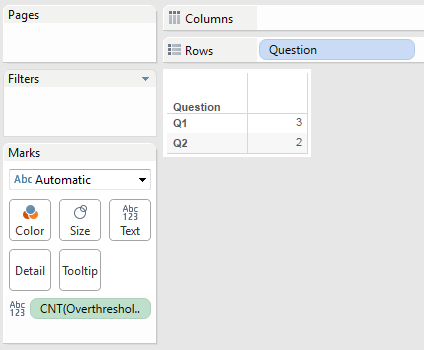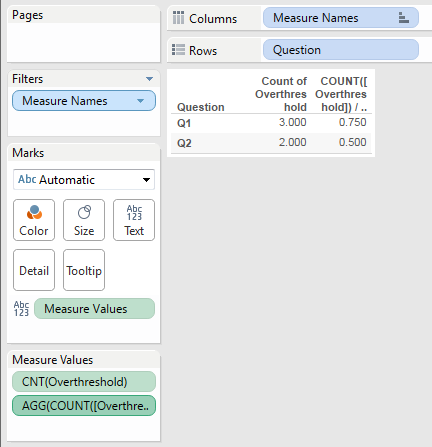# How to count occurrence of value and percentage of a subset in tableau public?

1 view
in BI

I have a set of data in the following format:

Resp | Q1 | Q2

P1   | 4  | 5

P2   | 1  | 2

P3   | 4  | 3

P4   | 6  | 4

I'd like to show the count and % of people who gave an answer greater than 3. So in this case, the output would be:

Question | Count  | Percent

Q1       |  3     | 75%

Q2       |  2     | 50%

Any suggestions?

by (47.2k points)
• As your data is not row-data based so you will have to pivot it.

• In the DataSource Screen choose column Q1 and Q1, right-click on them and choose "Pivot"

• You have to name the column with the answers "Answers" (just for clarity. You should get a table that looks like this:• Now you need to create a calculated field (I called it Overthreshold to check for your condition:

• Now, you could substitute the 3 with a parameter in case you want to easily change that condition. You can already drop the pills as follows to get the count:• Now if you want the percentage it gets a bit more complicated since you have to determine the count of the questions and the count of the answers > 3 which is information that is stored in two different columns.

• Create another calculated field with this calculation COUNT([Overthreshold]) / AVG({fixed [Question]:count([Answer])})

• Now, drop the created pill onto the "text" field or into the columns drawer and see the percentage values

• right-click on the field and choose Default Properties / Number Format to have it as a percentage rather than a float• Let's see what the formula does. It takes the count of the answers that are over the threshold and divides it by the count of answers for each question. This is done by the fixed part of the formula which counts the rows that have the same value in the Question column.

• The AVG is only there because Tableau needs an aggregation there. Since the value will be the same for every record of the question, you could also use MIN or MAX.